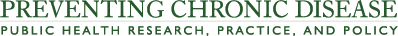# Effect of Vitamin D on Blood Pressure and Hypertension in the General Population: An Update Meta-Analysis of Cohort Studies and Randomized Controlled Trials

PEER REVIEWED

Figure 1.

Nonlinear dose–response association between circulating 25(OH)D levels and hypertension risk, update meta-analysis of cohort studies of the effect of 25(OH)D levels on hypertension in the general population. The dashed line indicates the pooled restricted cubic spline model, and the solid lines indicate the 95% CIs of the pooled curve. Abbreviations: 25(OH)D, 25-hydroxyvitamin D; CI, confidence interval.

Figure 1. Nonlinear dose–response association between circulating 25(OH)D levels and hypertension risk, update meta-analysis of cohort studies of the effect of 25(OH)D levels on hypertension in the general population. The dashed line indicates the pooled restricted cubic spline model, and the solid lines indicate the 95% CIs of the pooled curve. Abbreviations: 25(OH)D, 25-hydroxyvitamin D; CI, confidence interval.
Circulating 25(OH)D levels, nmol/L Relative risk (95% CI)
30 1.26 (1.10–1.43)
50 1.10 (1.01–1.16)
75 1 [Reference]
90 0.98 (0.96–1.00)
110 0.94 (0.89–1.00)

Figure 2.

Meta-analysis of effect of vitamin D supplementation on systolic blood pressure, update meta-analysis of randomized controlled trials of the effect of vitamin D on blood pressure in the general population. Abbreviations: CI, confidence interval; WMD, weighted mean difference.

Meta-analysis of effect of vitamin D supplementation on systolic blood pressure, update meta-analysis of randomized controlled trials of the effect of vitamin D on blood pressure in the general population. Abbreviations: CI, confidence interval; WMD, weighted mean difference.
Study (year) WMD, systolic blood pressure, mm Hg (95% CI) Weighted %
Bislev et al (2018) 0.50 (−2.97 to 3.97) 4.20
Tomson et al (2017) −2.50 (−6.76 to 1.76) 2.78
Sluyter et al (2017) −2.10 (−4.98 to 0.78) 6.11
Seibert et al (2017) −0.90 (−3.48 to 1.68) 7.62
McMulllan et al (2017) 0.20 (−2.89 to 3.29) 5.28
Bressendorff et al (2016) 1.90 (−0.93 to 4.73) 6.33
Mitchell et al (2015) 0 (−3.74 to 3.74) 3.61
Scragg et al (2014) −0.30 (−2.82 to 2.22) 7.93
Moghassemi et al (2014) −1.89 (−9.18 to 5.40) 0.95
Ramly et al (2014) −0.80 (−4.52 to 2.92) 3.65
Gagnon et al (2014) 4.62 (−0.51 to 9.75) 1.92
Forman et al (2013) −5.70 (−11.52 to 0.12) 1.49
Witham et al (2013) 3.00 (−1.77 to 7.77) 2.23
Wamberg et al (2013) −5.00 (−12.89 to 2.89) 0.81
Toxqui et al (2013) −4.00 (−7.23 to −0.77) 4.86
Zhu et al (2013) 1.50 (−3.60 to 6.60) 1.94
Gepner et al (2012) 2.20 (−1.44 to 5.84) 3.82
Wood et al (2012) 0.90 (−2.16 to 3.96) 5.41
Salehpour et al (2012) 2.71 (−2.43 to 7.85) 1.91
Maki et al (2011) 3.00 (−0.32 to 6.32) 4.58
Jorde et al (2010) 2.30 (−0.86 to 5.46) 5.06
Nagpal et al (2009) 3.69 (−0.49 to 7.87) 2.89
Daly et al (2009) 1.50 (−2.31 to 5.31) 3.47
Zittermann et al (2009) −1.00 (−5.88 to 3.88) 2.12
Major et al (2007) −2.50 (−6.06 to 1.06) 4.00
Pfeifer et al (2001) −7.40 (−12.31 to −2.49) 2.10
Scragg et al (1995) 0 (−4.16 to 4.16) 2.92
Overall (I2, 41.7%; P = .01) 0 (−0.71 to 0.71) 100.0

Figure 3.

Meta-analysis of effect of vitamin D supplementation on diastolic blood pressure, update meta-analysis of randomized controlled trials of the effect of vitamin D on blood pressure in the general population. Abbreviation: WMD, weighted mean difference.

Meta-analysis of effect of vitamin D supplementation on diastolic blood pressure, update meta-analysis of randomized controlled trials of the effect of vitamin D on blood pressure in the general population. Abbreviation: WMD, weighted mean difference.
Study (year) WMD, diastolic blood pressure, mm Hg (95% CI) Weighted %
Bislev et al (2018) 2.00 (−2.05 to 6.05) 1.39
Tomson et al (2017) −0.80 (−3.42 to 1.82) 3.32
Sluyter et al (2017) −0.80 (−2.48 to 0.88) 8.13
Seibert et al (2017) −0.40 (−2.44 to 1.64) 5.48
McMulllan et al (2017) −0.60 (−2.91 to 1.71) 4.28
Bressendorff et al (2016) 2.20 (0.35 to 4.05) 6.68
Mitchell et al (2015) −1.00 (−4.33 to 2.33) 2.06
Scragg et al (2014) 0.70 (−1.05 to 2.45) 7.43
Moghassemi et al (2014) −3.72 (−8.35 to 0.91) 1.07
Ramly et al (2014) −0.22 (−2.93 to 2.49) 3.11
Gagnon et al (2014) 1.36 (−2.96 to 5.68) 1.22
Forman et al (2013) −2.50 (−6.80 to 1.80) 1.24
Witham et al (2013) 0.60 (−2.46 to 3.66) 2.44
Wamberg et al (2013) −4.00 (−9.38 to 1.38) 0.79
Toxqui et al (2013) −2.20 (−4.84 to 0.44) 3.27
Zhu et al (2013) −1.90 (−5.44 to 1.64) 1.82
Gepner et al (2012) −0.30 (−2.08 to 1.48) 7.20
Wood et al (2012) 1.20 (−0.26 to 2.66) 10.73
Salehpour et al (2012) 2.17 (−1.20 to 5.54) 2.00
Maki et al (2011) 1.90 (−0.45 to 4.25) 4.12
Jorde et al (2010) 0.80 (−1.25 to 2.85) 5.42
Nagpal et al (2009) 1.54 (−1.81 to 4.89) 2.03
Daly et al (2009) 0.30 (−2.46 to 3.06) 2.99
Zittermann et al (2009) 0 (−2.90 to 2.90) 2.71
Major et al (2007) 0 (−2.67 to 2.67) 3.20
Pfeifer et al (2001) −0.30 (−3.40 to 2.80) 2.38
Scragg et al (1995) 0 (−2.57 to 2.57) 3.47
Overall (I2, 3.3%; P = .42) 0.19 (−0.29 to 0.67) 100.0